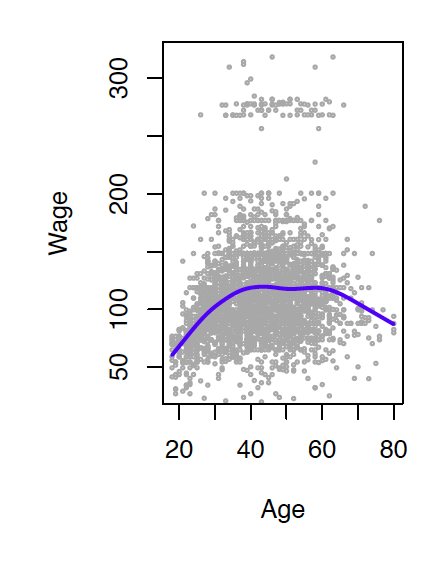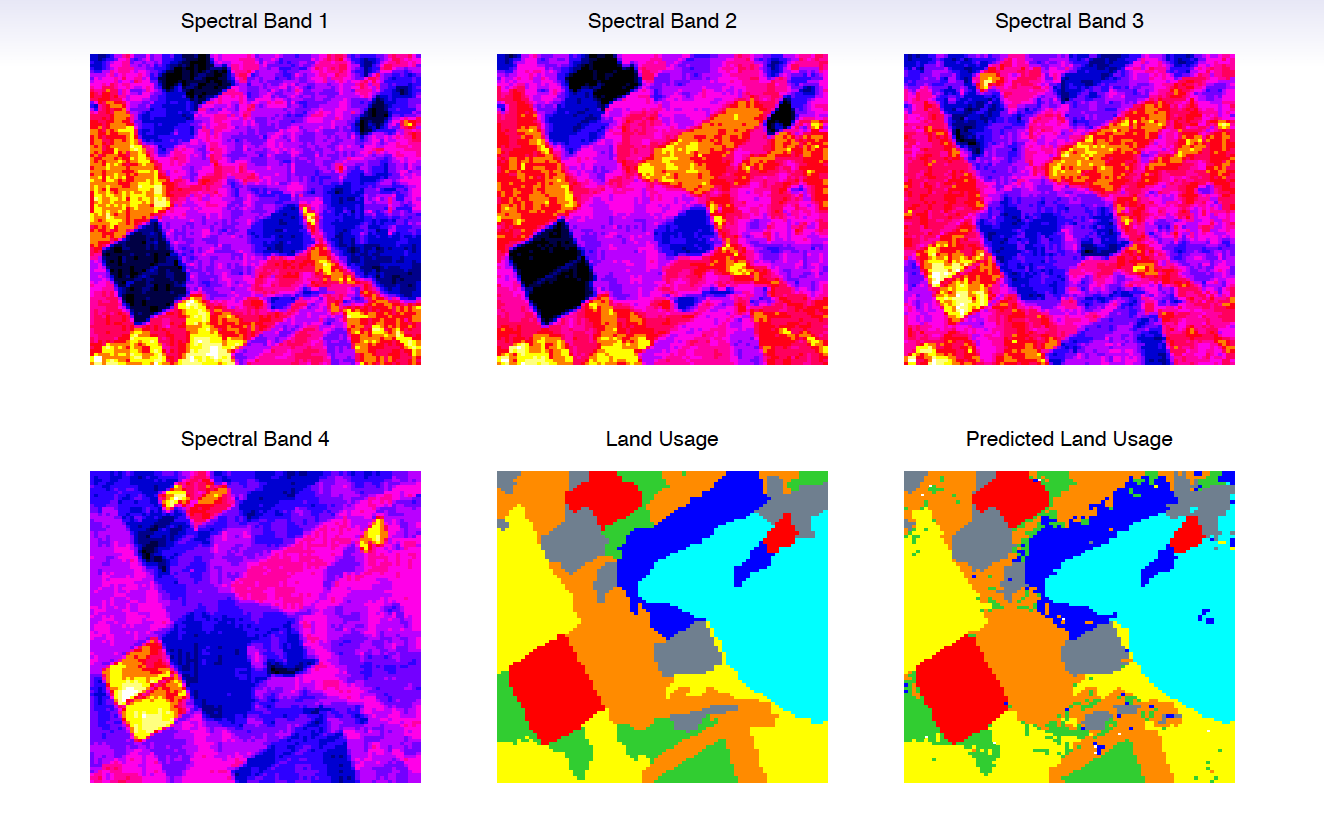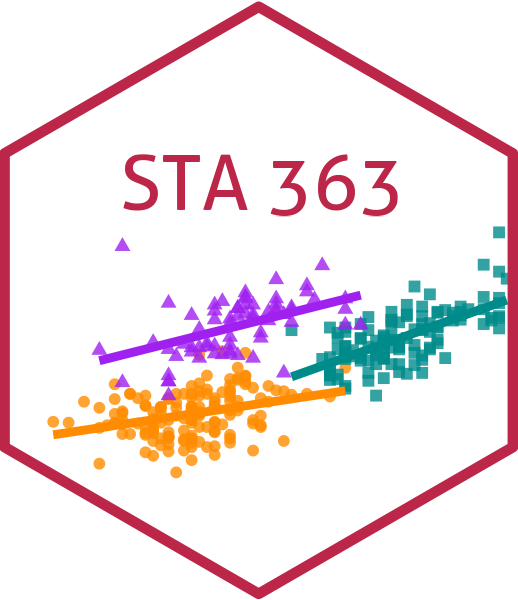# Welcome to Statistical Learning

Dr. D’Agostino McGowan

## 👋

### Lucy D’Agostino McGowan

mcgowald@wfu.edu
Thurs 10a-11a

# bit.ly/sta-363-s23

## Intros

• Name
• Major
• Fun OR boring fact

## Statistical Learning Problems

• Identify risk factors for breast cancer

## Statistical Learning Problems

• Identify risk factors for breast cancer
• Customize an email spam detection system
• Data: 4601 labeled emails sent to George who works at HP Labs
• Input features: frequencies of words and punctuation
george you hp free ! edu remove
spam 0.00 2.26 0.02 0.52 0.51 0.01 0.28
email 2.27 1.27 0.90 0.07 0.11 0.29 0.01

## Statistical Learning Problems

• Identify risk factors for breast cancer
• Customize an email spam detection system
• Identify numbers in handwritten zip code

# DEMO

## Statistical Learning Problems

• Identify risk factors for breast cancer
• Customize an email spam detection system
• Identify numbers in handwritten zip code
• Establish the relationship between variables in population survey data

Income survey data for males from the central Atlantic region of US, 2009# Statistical Learning Problems

• Identify risk factors for breast cancer
• Customize an email spam detection system
• Identify numbers in handwritten zip code
• Establish the relationship between variables in population survey data
• Classify pixels of an imageUsage $\in$ {red soil, cotton, vegetation stubble, mixture, gray soil, damp gray soil}

## ✌️ types of statistical learning

• Supervised Learning
• Unsupervised Learning

## Supervised Learning

• outcome variable: $Y$, (dependent variable, response, target)
• predictors: vector of $p$ predictors, $X$, (inputs, regressors, covariates, features, independent variables)
• In the regression problem, $Y$ is quantitative (e.g price, blood pressure)
• In the classification problem, $Y$ takes values in a finite, unordered set (survived/died, digit 0-9, cancer class of tissue sample)
• We have training data $(x_1, y_1), \dots, (x_N, y_N)$. These are observations (examples, instances) of these measurements

## Supervised Learning

What do you think are some objectives here?

### Objectives

• Accurately predict unseen test cases
• Understand which inputs affect the outcome, and how
• Assess the quality of our predictions and inferences

## Unsupervised Learning

• No outcome variable, just a set of predictors (features) measured on a set of samples
• objective is more fuzzy – find groups of samples that behave similarly, find features that behave similarly, find linear combinations of features with the most variation
• difficult to know how well your are doing
• different from supervised learning, but can be useful as a pre-processing step for supervised learning

## Let’s take a tour - class website

• Concepts introduced:
• How to find slides
• How to find assignments
• How to find RStudio Cloud
• How to get help
• How to find policies